Chapter 8

The Problem of Plateau

Another principle that operates throughout the known Universe is that spheres and circular patterns are everywhere. We find them at the atomic level, in raindrops, in the shape of some bacteria, peas, soap bubbles, the Earth, the planets, the orbits of planets, the stars, the shape of galaxies, and even in the shape of highs and lows on the surface of the Earth.

What are the common links between matter, and the space that matter occupies, that leads to the circular or spherical patterns and structures?

One common thread that runs through all phenomena in the Universe is that the sphere has the least surface area for a given volume and is the most suitable structure for restraining internal forces. The exact nature of the forces are immaterial, since they can be nuclear, electrostatic, magnetic, surface tension, elasticity, hydrostatic, centrifugal, convective, gravitational, etc. The type of forces that operate in any given structure are determined partly by the size, amount, and the kind of matter, in a given amount of space. For examples: man is controlled by gravitation; a water beetle by the surface tension on the surface of a pool of water; while bacteria are controlled by the viscosity of the fluid, Brownian movement, and electrostatic charges. The forces controlling high and low pressure centers are usually expressed as pressure differences, centrifugal, gravitational, convective, coriolis forces, etc.

The Conic Sections

The geometrical pattern of a sphere or a circle becomes lost from time to time due to the unbalancing of the forces that would normally lead to a spherical shape. To what new forms do unbalanced spherical patterns

### 82

change? They often change to shapes or curves known as conic sections, which were discovered in the fourth century B. C., by Menaechmus, and more completely investigated by Apollonius in the third century B. C. These conic sections are formed by the intersection of a plane and a right circular cone (a solid generated by rotating a right triangle around one of the legs of the 90° angle). See Figure 8-1, which shows a double cone.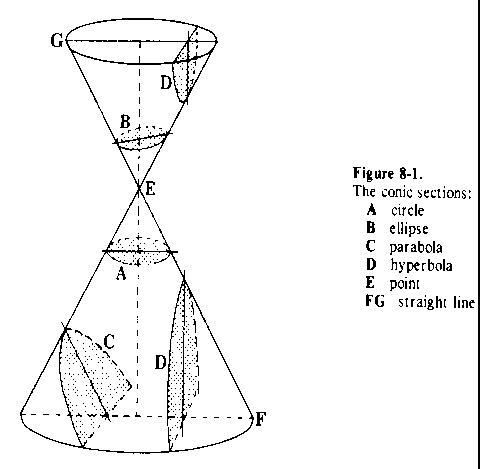If a plane is sliced through the cone in such a way that the plane is perpendicular to the axis of the cone, we get a section of the cone that is a circle (Figure 8-1 A). If the plane cuts the circular cone obliquely to the axis, we get a section of the cone that is an ellipse (Figure 8-1 B). If the cutting plane is parallel to one of the sides of the circular cone, we get a parabola (Figure 8-1 C). If the plane intersects each of the two cones, we get a hyperbola (Figure 8-1 D). If the cutting plane is tangent to one of the sides of the circular cone, we get a straight line (Figure 8-1 EF). And if the cutting plane is outside the cone, but touches at point E, we get a point (Figure 8-1 E).

The mathematics and other details of these shapes are explained in any elementary book on geometry. All we want to establish at this time is that a structure with the shape of any given one of the conic section can be transformed into a different kind of conic section under the

### 83

imposition of proper deforming forces. For example: a hyperbolic shape can drift into a parabolic shape; a parabolic shape into an elliptical one; an elliptical into a circular, and a circular into a dot; and many other combinations. Are we far afield from the weather map? Of course not! We are laying the groundwork for analyzing the myriad of configurations that highs and lows take on the surface of the Earth.

Surfaces of Revolution

We now come to Plateau's surfaces of revolution, which are surfaces of objects that are symmetrical around an axis. They represent examples of other shapes into which conic sections can be transformed. These other shapes, inevitably, are involved in the shapes of various weather phenomena from the size of huge storms to the size of small clouds. These principles were discovered in 1873 by Joseph Plateau. He found that there are only 6 types of symmetrical surfaces that can be formed by oil globules or soap bubbles. A bubble can be blown up into a sphere, or shaped into a plane surface, cylinder, unduloid, catenoid, or nodoid. See Figure 8-2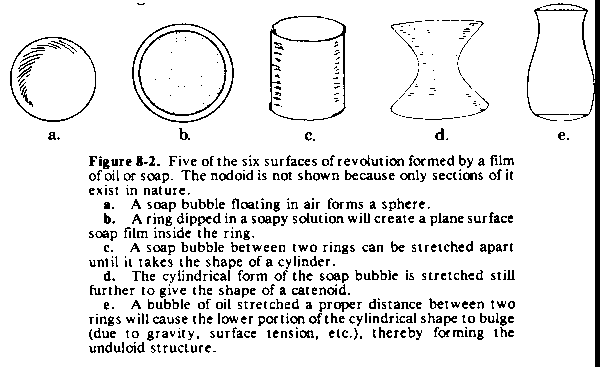.

All these surfaces are related and can easily slip from one to another. The mathematical relationship between them was first shown by Delaunay (8) in 1841. He proved that the mathematical curves shown in Figure 8-3, which are generated as roulettes of the conic sections, can be used to create the shapes of Figure 8 2.

### 84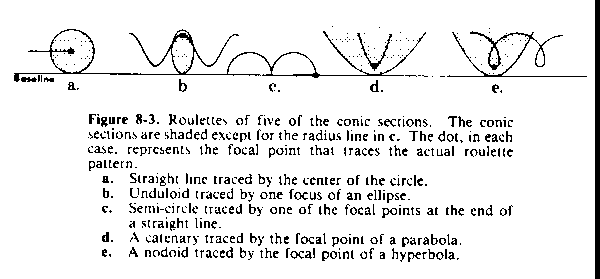Take two curves lying in the same plane. Assume one of the curves to be fixed in position (in Figure 8-3, the baseline is considered to be the fixed curve). The curve that moves is rolled (no sliding permitted) on the fixed curve. Any point connected with the rolling curve describes a curve called a roulette. If we roll a circle, an ellipse, a parabola, a hyperbola, and a short straight line on the baseline, we will get the forms shown in Figure 8-3.

If we roll an ellipse on the baseline, either one of its foci will trace a wavy line which is the unduloid--in the figure, we show the curve formed by only one of the foci.

As can be seen in Figure 8-4, every ellipse has two axes (one axis passes through the two focal points, with the other axis at right angles to it and passing through the center of the ellipse). We find that the more unequal the length of these axes, the greater will be the swings of the undulation of the roulette. If the two axes are equal, the ellipse becomes a circle. The curve created by the rolling center of a circle is a straight line parallel to the line on which it is rolling. This roulette line, when rotated around the baseline, creates a cylinder. So we can see that the unduloid changes to a cylinder when the ellipse reduces to a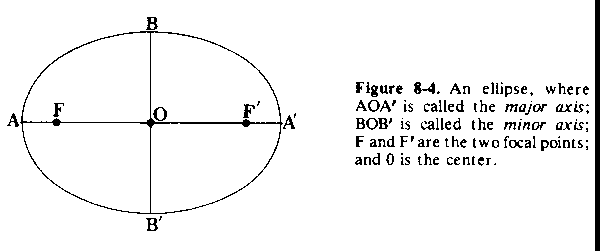### 85

circle. If one axis of the ellipse decreases to zero length but the other axis still has a definite length, then the ellipse is reduced to a straight line with one focus at each end. This generates a roulette of semicircles. These semicircles, in turn, rotate around the baseline to create a series of spherical surfaces.

Collapse of Cylindrical Structures

Plateau also proved that a cylinder of liquid is not a structure of stable equilibrium if its length is longer than the circumference (about 3.14 times its diameter). A long cylindrical liquid rod, like in Figure 8-5, if it could be assembled and then left by itself, would immediately collapse into a row of equally sized and equally spaced drops. There is a very simple law that the distance between the centers of the drops is equal to the circumference of the cylinder.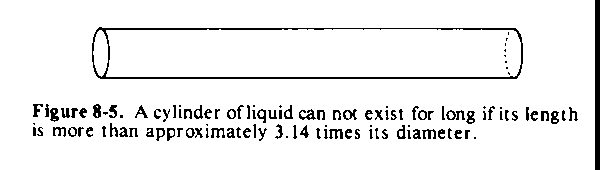In the above example, the cause of collapse would be due, mainly, to surface tension. Surface tension is that property which causes the surface film of all liquids to try and take a form which has the least surface area; in other words, a sphere.

Plateau was able to show by his experiments that any disturbances formed at distances less than the circumference of the cylinder would result in a configuration as in Figure 8-6. The disturbance would affect the curvature of the outside surface in such a way as to make the surface tension push the bulges (shown in the figure) back and pull the hollows outwardly. What if the bulges are farther apart than the circumference of the cylinder? Then the sharper curvature of the skinnier parts will force the liquid in the skinnier part into the parts that are already wider. Thus any such disturbance would cause the bulges to separate still farther, or cause the whole thing to break into drops. Before the breakup occurs, the drops are joined by narrow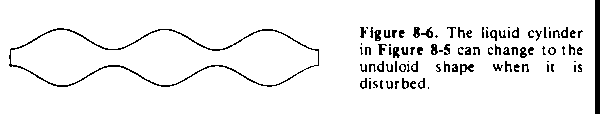### 86

necks of liquid (Figure 8-7a). These narrow necks split themselves up into smaller secondary droplets (Figure 8-7b). When dealing with different substances, there will be variations in the actual results obtained. These variations are determined by such things as the viscosity, the inertia of the fluid, etc.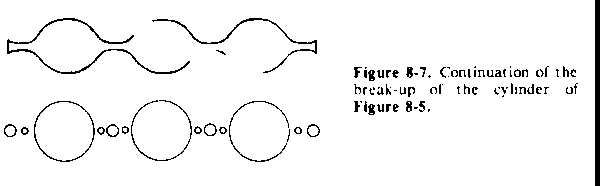Plateau explains the formation of the smaller beads as follows: When contact is nearly broken between two big spheres, there is still a narrow thin neck. The fluid does not flow easily out of this neck into the larger spheres because of internal friction. This thin cylinder now acts like another cylinder, but of smaller circumference. Therefore, it also breaks up when the length becomes greater than the new, smaller circumference. The length is usually such that it breaks at two points. This leaves an end piece attached to each sphere, which is absorbed, and a small sphere in the center. Sometimes the same process of formation of a connecting thread is repeated a second time between the small intermediate bead and the larger sphere. In this case, there are two additional beads of still smaller size on each side of the first small one. This whole process can be observed in a spider web. The thread of a web is formed from a secretion of the spider's glands. It comes out as a semifluid cylinder which breaks up into little connected beads which then harden. See Figure 8-8 for an enlarged view of a strand of spider web.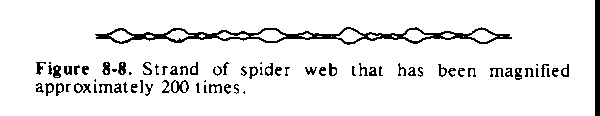This process can also be seen in the breaking of a wave against a seashore. As the wave approaches the shoreline, it presents a long, smooth horizontal cylindrical edge. This cylindrical edge breaks up at a given moment into an array of little jets which then subsequently break up into foam. At the same time, we find that the back of the wave which was previously smooth, will have ridges and gullies. The jets are

### 87

caused by the breakup of the cylindrical edge, as explained by Plateau, while the ridges between the gullies mark the lines of easier flow determined by the position of the jets. See Figure 8-9.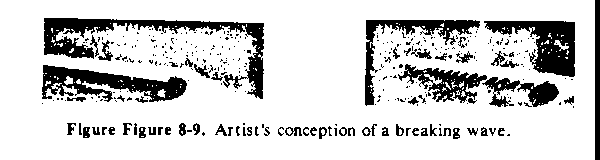You can take a piece of glass tubing; heat it, and then draw it out. You will form an unduloid portion as in Figure 8-l0a. If you blow on the other end, you will form another unduloid of opposite curvature as in Figure 8-10b.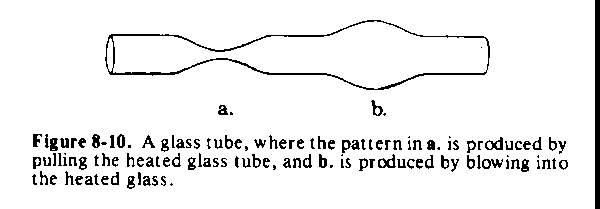Wilhelm F. Hofmeister (1824-1877) (9) dipped the long root hairs of a water plant, such as Trianea, in a denser fluid containing glycerine. The sap of the cells would diffuse outwards; the protoplasm would separate from the surrounding wall, and then lie loose as a cylinder of protoplasm inside the cell. Soon thereafter, it would show signs of instability and break up into little spherical globules, as shown in Figure 8-11. The narrow surroundings of the spherules prevent them from taking a completely spherical form, giving instead the unduloid pattern trying to separate into spheres. In between, we have the relatively regular beads of smaller size.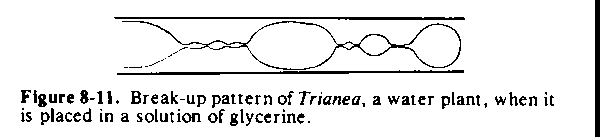Spheres, cylinders, and unduloids are the most common shapes among the forms of small single celled organisms-in the processes of growth and decay, they readily transform from one form to another. Figure 8-12 shows some examples.

### 88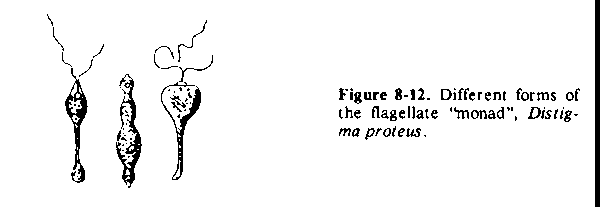The sphere encloses the greatest volume with the least surface area if there are no outside constraints. On the other hand, some other Plateau configurations are subject to outside constraints, which results in different shapes for the surfaces of minimum area (other than the sphere). These outside constraints may be caused by a pipe which supports a soap bubble; in the case of cells, by partial or complete hardening of the cell walls or surfaces; etc. Joseph Louis Lagrange (1736-1813) developed the formula for finding a minimal surface for the boundary of any given closed curve in space. This formula is referred to as the problem of Plateau, who solved it with his soap films in 1873.

The Splash of a Drop

This brings us to A. M. Worthington (10) and his study of splashes. He points out that what Plateau developed for a straight cylinder of liquid also applies if this straight cylinder is bent into a ring. This ring will break up into a ring of drops.

In another article (11), he showed a series of drawings representing the splash of a drop of mercury 0.15 inch in diameter that fell 3 inches onto a smooth glass plate. Figure 8-13 is a selection of these drawings that show the progression from a single drop to a final smaller drop in the center surrounded by 12 still smaller drops separated equally from each other at an angle of 30°. The significant feature from the standpoint of weather, is the orderly and systematic angular and circumferential arrangement of the rays (and finally droplets) around the center of the splash.

The Center of a Structure is Related to its Shape

In another area, we find that Lord Rayleigh (1842-1919) (12) dealt with the Plateau phenomenon by analyzing its implications on the

### 89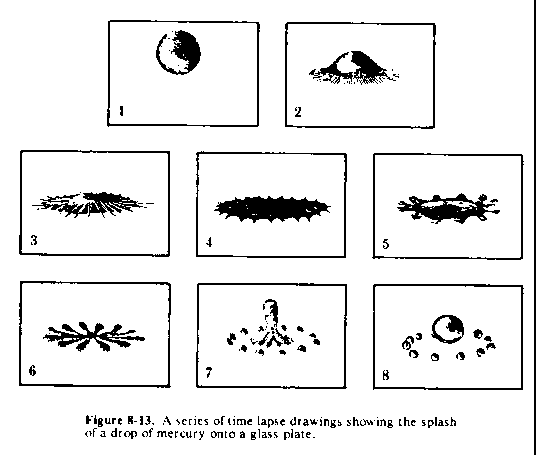stability and instability of turbulence. But no one has mentioned how this phenomenon applies to large scale patterns of high and low pressure centers over an entire hemisphere. Of course, there are many different forces involved, but recognizing the conic sections and Plateau's curves are helpful in understanding the shapes of highs and lows as seen on hemispheric weather maps. Locating the exact centers (or focal points) of highs and lows is extremely important, as will be seen in the many charts used to analyze what happened at 1230 GMT, 7 December 1950. Recognizing the type of shape of a vortex is an aid in locating its center. You must keep in mind that the true shape of the Plateau figures on the surface of the Earth are distorted on the polar stereographic map due to the projection.